# Measurement: Reading Weights From a Scale

In this worksheet, students learn about how to read a weight in kilograms and half kilograms from a scale or balance.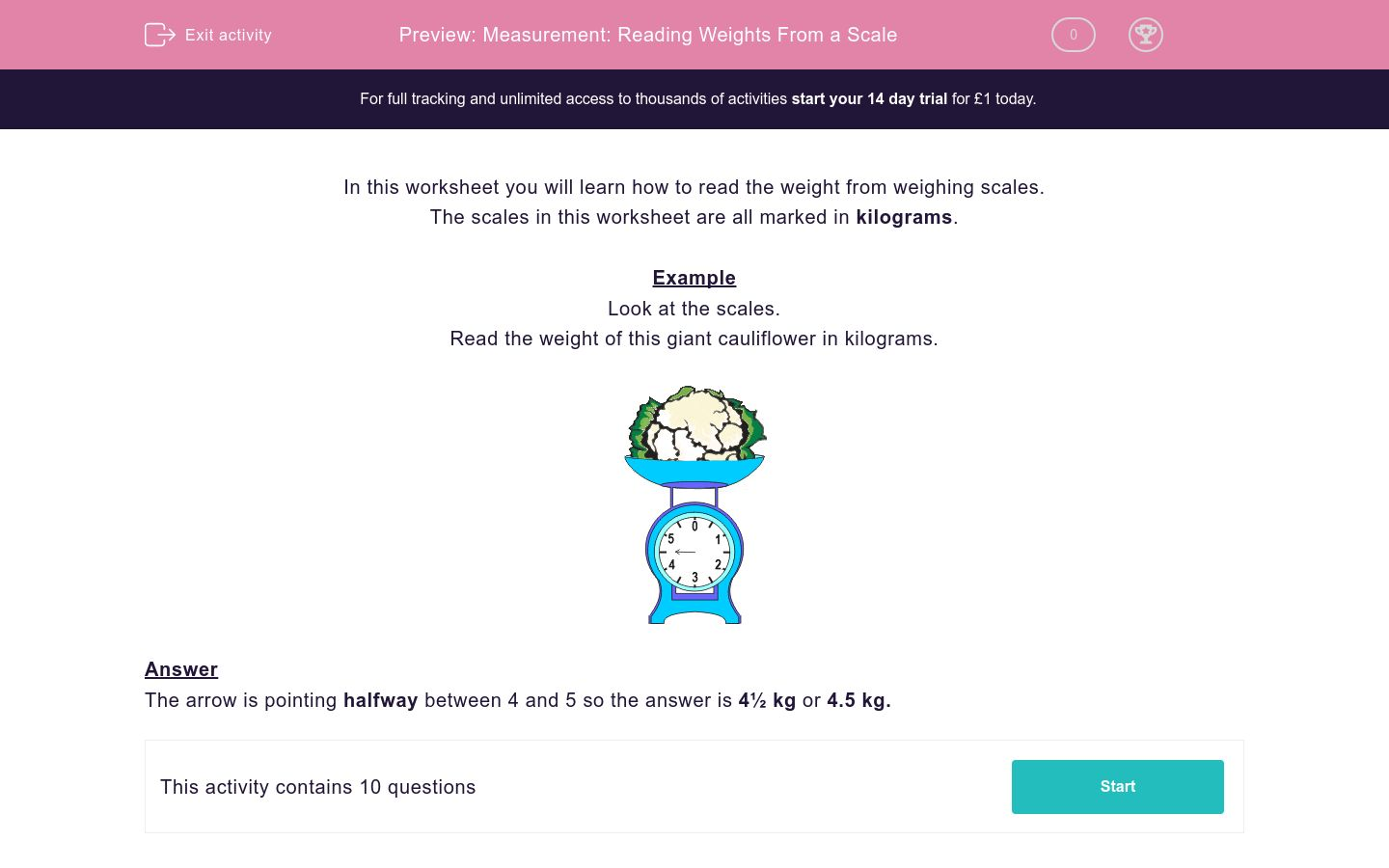Key stage:  KS 2

Curriculum topic:   Maths and Numerical Reasoning

Difficulty level:### QUESTION 1 of 10

In this worksheet you will learn how to read the weight from weighing scales.

The scales in this worksheet are all marked in kilograms.

Example

Look at the scales.

Read the weight of this giant cauliflower in kilograms.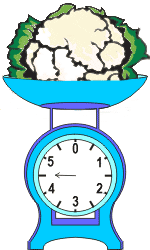The arrow is pointing halfway between 4 and 5 so the answer is 4½ kg or 4.5 kg.

Look at the scales.

Read the weight of this object in kilograms.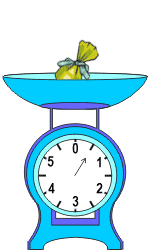1 kg

500 kg

½ kg

Look at the scales.

Read the weight of these objects in kilograms.5 kg

6 kg

3 kg

Look at the scales.

Read the weight of this object in kilograms.25 kg

2½ kg

250 kg

Look at the scales.

Read the weight of this object in kilograms.1 kg

3 ½ kg

½ kg

Look at the scales.

Read the weight of this object in kilograms.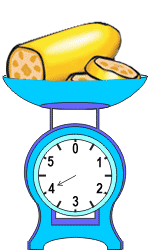3 kg

4½ kg

4 kg

Look at the scales.

Read the weight of these objects in kilograms.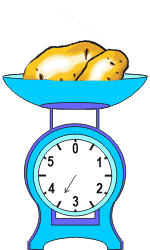3½ kg

35 kg

3 kg

Look at the scales.

Read the weight of this object in kilograms.½ kg

1½ kg

1 kg

Look at the scales.

Read the weight of this object in kilograms.2 g

1½ kg

2 kg

Look at the scales.

Read the weight of this object in kilograms.½ kg

5½ kg

6 kg

Look at the scales.

Read the weight of this object in kilograms.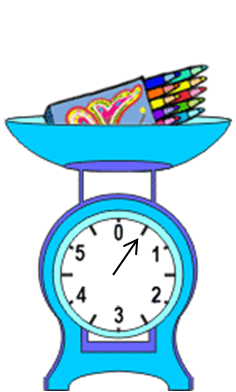half a kg

0.5 kg

15 kg

1.5 kg

½ kg

• Question 1

Look at the scales.

Read the weight of this object in kilograms.½ kg
EDDIE SAYS
The arrow is pointing halfway between 0 and 1.
• Question 2

Look at the scales.

Read the weight of these objects in kilograms.3 kg
EDDIE SAYS
The arrow is pointing exactly to 3.
• Question 3

Look at the scales.

Read the weight of this object in kilograms.2½ kg
EDDIE SAYS
The arrow is pointing halfway between 2 and 3.
• Question 4

Look at the scales.

Read the weight of this object in kilograms.1 kg
EDDIE SAYS
The arrow is pointing exactly to 1.
• Question 5

Look at the scales.

Read the weight of this object in kilograms.4 kg
EDDIE SAYS
The arrow is pointing exactly to 4.
• Question 6

Look at the scales.

Read the weight of these objects in kilograms.3½ kg
EDDIE SAYS
The arrow is pointing halfway between 3 and 4.
• Question 7

Look at the scales.

Read the weight of this object in kilograms.1 kg
EDDIE SAYS
The arrow is pointing exactly to 1.
• Question 8

Look at the scales.

Read the weight of this object in kilograms.2 kg
EDDIE SAYS
The arrow is pointing exactly to 2.
• Question 9

Look at the scales.

Read the weight of this object in kilograms.5½ kg
EDDIE SAYS
The arrow is pointing half a mark past the 5.
• Question 10

Look at the scales.

Read the weight of this object in kilograms.half a kg
0.5 kg
½ kg
EDDIE SAYS
The arrow is pointing halfway between 0 and 1.
All of these are a half.
---- OR ----

Sign up for a £1 trial so you can track and measure your child's progress on this activity.

### What is EdPlace?

We're your National Curriculum aligned online education content provider helping each child succeed in English, maths and science from year 1 to GCSE. With an EdPlace account you’ll be able to track and measure progress, helping each child achieve their best. We build confidence and attainment by personalising each child’s learning at a level that suits them.

Get started